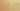VariablesHoldExpressions

Misconception:

For example, a = v/2 will store the expression v/2 (or a reference to the expression v/2) in the variable a.Incorrect

= stores an expression: it stores a reference to the expression in a variable

Correct

= evaluates an expression and stores a reference to its value in a variable

CorrectionHere is what's right.

First, the expression is evaluated. A reference to the resulting value is stored in the variable.

In Python, variables reference values. A non-evaluated expression is not a value. Thus, variables cannot reference non-evaluated expressions. Instead, the expression is evaluated, producing a value.

Once a value is produced, there is no longer a connection between the value and the expression that produced it.

OriginWhere could this misconception come from?

This misconception may at least partially be due to students’ familiarity with algebra, where the right hand side of an equation is not evaluated and stored in a variable on the left.

While chances are low, it could be that a student got exposed to “pass-by-name” beforehand.

SymptomsHow do you know your students might have this misconception?

A student holding this misconception may put the expression (not a reference to its value) into the box of a memory diagram.

ValueHow can you build on this misconception?

This misconception can be very valuable when introducing functional programming, where functions (including anonymous functions, lambdas) are treated as normal values.

If a student (wrongfully) thinks that the following statement stores a reference to the unevaluated expression a + 1 in variable inc

inc = a + 1

… then when introducing lambdas one can explain that the following statement does do that:

inc = lambda a : a + 1

Python